# Mental Ability, Analytical & Logical Reasoning- Solved Questions(1995-2020)- 1 Notes | Study UPSC Topic Wise Previous Year Questions - UPSC

## UPSC: Mental Ability, Analytical & Logical Reasoning- Solved Questions(1995-2020)- 1 Notes | Study UPSC Topic Wise Previous Year Questions - UPSC

The document Mental Ability, Analytical & Logical Reasoning- Solved Questions(1995-2020)- 1 Notes | Study UPSC Topic Wise Previous Year Questions - UPSC is a part of the UPSC Course UPSC Topic Wise Previous Year Questions.
All you need of UPSC at this link: UPSC

Question 1: What is the missing term @ in the following?
ACPQ : BESU :: MNGI : @        [2020-II]
(a) NPJL
(b) NOJM
(c) NPIL
(d) NPJM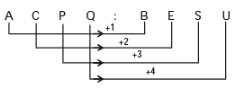Similarly,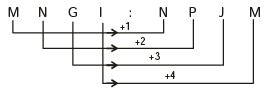Question 2: The letters from A to Z are numbered from 1 to 26 respectively. If GHI = 1578 and DEF = 912, then what is ABC equal to:    [2020-II]
(a) 492
(b) 468
(c) 262
(d) 246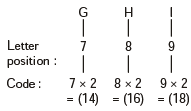⇒ 14(16)(18) = (14)(16 + 1)8 = (14 + 1)78= 1578.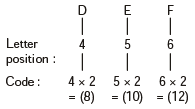⇒ 8(10)(12) = 8(10 + 1)(2) = (8 + 1)(1)(2)= 912.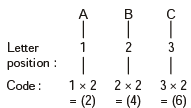⇒ 246.

Question 3: Consider the following statements:
1. The minimum number of points of intersection of a square and a circle is 2.
2. The maximum number of points of intersection of a square and a circle is 8.
Which of the above statements is/are correct?    [2020-II]

(a) 1 only
(b) 2 only
(c) Both 1 and 2
(d) Neither 1 nor 2

(1) Minimum number of point of intersection of a square and a circle = 0.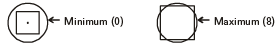(2) The maximum number of point of intersection of a square and circle = 8.
Hence, (1) is not correct but (2) is correct.

Question 4: If in a particular year 12th January is a Sunday, then which one of the following is correct?    [2020-II]
(a) 15th July is a Sunday if the year is leap year.
(b) 15th July is a Sunday if the year is not a leap year.
(c) 12th July is a Sunday if the year is a leap year.
(d) 12th July is not a Sunday if the year is a leap year.

Number of days between 12th January to 12th July in a leap year.
= 19 + 29 + 31 + 30 + 31 + 30 + 12 = 182 = 26 weeks
Hence, 12th July will be same as 12th January in a leap year. So, it will be Sunday.

Question 5: A family of two generations consisting of six members P, Q, R, S, T and U has three males and three females. There are two married couples and two unmarried siblings. U is P’s daughter and Q is R’s mother-in-law. T is an unmarried male and S is a male. Which one of the following is correct?     [2020-II]
(a) R is U’s husband.
(b) R is S’s wife.
(c) S is unmarried.
(d) None of the above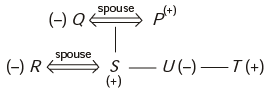[Here, (+) - Male, (–) - Female]
In the family of 6 members, three are males and three are females. Now, when R is U's husband, then number of male members becomes 4 i.e. T, S, R and P.
So, R must is a female and daughter-in-law of Q. Q is the wife of P. T is an unmarried brother of P. U is unmarried sister of S.

Question 6: Two Statements S1 and S2 are given below followed by a Question:
S1: There are not more than two figures on any page of a 51-page book.
S2: There is at least one figure on every page.
Question:
Are there more than 100 figures in that book?
Which one of the following is correct in respect of the above Statements and the Question?    [2020-II]

(a) Both S1 and S2 are sufficient to answer the Question, but neither S1 alone nor S2 alone is sufficient to answer the Question.
(b) S1 alone is sufficient to answer the Question.
(c) S1 and S2 together are not sufficient to answer the Question.
(d) S2 alone is sufficient to answer the Question.

From S1 and S2, we get
1 ≤ Number of figures on each page ≤ 2
∴ 51 ≤ Number of figures on 51 pages ≤ 102
Hence, we can not get exact number of figures on 51 pages by the given two statements.

Question 7: Consider the following arrangement that has some missing letters:
abab_b_bcb_dcdcded_d
The missing letters which complete the arrangement are    [2020-II]
(a) a, b, c, d
(b) a, b, d, e
(c) a, c, c, e
(d) b, c, d, e

The missing letter's that complete the series are :
a b a b b b c b d c d c d e d e d
The pattern is ababa, bcbcb, cdcdc, deded
Hence, letters a, c, c, e complete the sequence.

Question 8: Two Statements S1 and S2 are given below with regard to four numbers P, Q, R and S followed by a Question:
S1: R is greater than P as well as Q.
S2: S is not the largest one.
Question:
Among four numbers P, Q, R and S, which one is the largest?
Which one of the following is correct in respect of the above Statements and the Question?    [2020-II]

(a) S1 alone is sufficient to answer the Question.
(b) S2 alone is sufficient to answer the Question.
(c) S1 and S2 together are sufficient to answer the Question, but neither S1 alone nor S2 alone is sufficient to answer the Question.
(d) S1 and S2 together are not sufficient to answer the Question.

From S1 : R > P and Q
From S2 : R > S, as S is not the largest one
From S1 and S2, R > P, Q and S
Hence, R is the largest.
Thus, both statements S1 and Stogether are sufficient to answer the question.

Question 9: Three Statements S1, S2 and S3 are given below followed by a Question:
S1: C is younger than D, but older than A and B.
S2: D is the oldest.
S3: A is older than B.
Question:
Who among A, B, C and D is the youngest?
Which one of the following is correct in respect of the above Statements and the Question?    [2020-II]
(a) S1 alone is sufficient to answer the Question.
(b) S1 and S2 together are sufficient to answer the Question.
(c) S2 and S3 together are sufficient to answer the Question.
(d) S1 and S3 together are sufficient to answer the Question.

From S1 : D > C > A, B
We get that D is oldest one among the given four persons.
From S3 : A > B, B is younger than A.
From S1 and S3, we get that,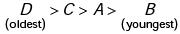B is the youngest.

Question 10: A is 16th from the left end in a row of boys and V is 18th from the right end. G is 11th from A towards the right and 3rd from V towards the right end. How many boys are there in the row?    [2020-II]
(a) 40
(b) 41
(c) 42
(d) Cannot be determined due to insufficient data

G's position from left end
= A's position from the left end + 11
= 16 + 11 = 27th from left end.
G's position from right end
= A's position from right end – 3
= 18 – 3 = 15th from right end.
Total number of boys in the row
= (27 + 15) – 1 = 41.

Question 11: Consider the following sequence of numbers :
5 1 4 7  3 9 8 5 7 2 6 3 1 5
8 6 3 8 5 2 2 4 3 4 9 6
How many odd numbers are followed by the odd number in above sequence?    [2020-II]
(a)5
(b) 6
(c)7
(d) 8

In the given series, there are 6 odd numbers that are followed by odd number.

Question 12: Two Statements are given followed by two Conclusions:
Statements:
All cats are dogs.
All cats are black.
Conclusion-I:
All dogs are black
Conclusion-II:
Some dogs are not black.
Which of the above Conclusions logically follows/follow from the two given Statements, disregarding commonly known facts?    [2020-II]
(a) Only Conclusion-I
(b) Only Conclusion-II
(c) Neither Conclusion-I nor Conclusion-II
(d) Both Conclusion-I and Conclusion-II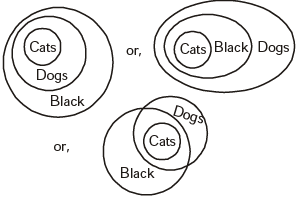Here, there are three conclusions we can draw from the given statements, thus neither conclusion-I nor conclusion II are true.

Question 13: A man walks down the backside of his house straight 25 metres, then turns to the right and walks 50 metres again; then he turns towards left and again walks 25 metres. If his house faces to the East, what is his direction from the starting point?    [2020-II]
(a) South -East
(b) South -West
(c) North-East
(d) North -West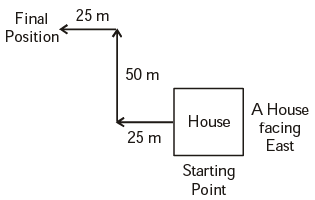Man's final position is in North-West with respect to his starting point.
When his house faces east, then man started towards the direction west from back of his house, after 25 m he turn to his right towards North direction, then after covering 50 m in the North-direction, he turns to his left and stopped at 25 m. Finally, he is towards North-West with respect to his starting point.

Question 14: Seeta and Geeta go for a swim after a gap of every 2 days and every 3 days respectively. If on 1st January both of them went for a swim together, when will they go together next?    [2019-II]
(a) 7th January
(b) 8th January
(c) 12th January
(d) 13th January

L.C.M of 3 and 4 = 12
together, they will meet on 1 + 12 = 13th jan.

Question 15: In a school, 60% students play cricket. A student who does not play cricket, plays football. Every football player has got a two-wheeler. Which of the following conclusions cannot be drawn from the above data?

1. 60% of the students do not have two-wheelers.
2. No cricketer has a two-wheeler.
3. Cricket players do not play football.
Select the correct answer using the code given below :
[2019-II]
(a) 1 and 2 only
(b) 2 and 3 only
(c) 1 and 3 only
(d) 1, 2 and 3

Every football player has got a two-wheeler does not mean that no cricketer has a two-wheeler.
So, we can not find the exact number of students do not have two-wheelers.
Again, Any Cricket player can also play Football.
Hence, conclusion (1), (2)  and (3) Can not be drawn from the data.

Question 16: P, Q and R are three towns. The distance between P and Q is 60 km, whereas the distance between P and R is 80 km. Q is in the West of P and R is in the South of P. What is the distance between Q and R?    [2019-II]
(a) 140 km
(b) 130 km
(c) 110 km
(d) 100 km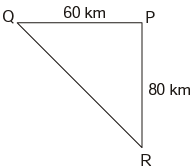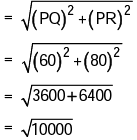= 100 km.

Question 17: A five-storeyed building with floors from I to V is painted using four different colours and only one colour is used to paint a floor.
Consider the following statements :
1. The middle three floors are painted in different colours.
2. The second (II) and the fourth (IV) floors are painted in different colours.
3. The first (I) and the fifth (V) floors are painted red.
To ensure that any two consecutive floors have different colours
(a) Only statement 2 is sufficient
(b) Only statement 3 is sufficient
(c) Statement 1 is not sufficient, but statement 1 along with statement 2 is sufficient
(d) Statement 3 is not sufficient, but statement 3 along with statement 2 is sufficient

From statement (3), floor 1 and 5 are painted by the same colour red.
Then, middle three floor 2, 3 and 4 must be painted in remaining three different colours.
Floor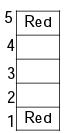In that way, we get that any two consticutive floor have different colours.

Question 18: Consider the following Statements and Conclusions:
Statements:
1. Some rats are cats.
2. Some cats are dog.
3. No dog is a cow.
Conclusions:
I. No cow is a cat.
II. No dog is a rat.
III. Some cats are rats.
Which of the above conclusions is/are drawn from the statements?    [2019-II]
(a) I, II and III
(b) Only I and II
(c) Only III
(d) Only II and III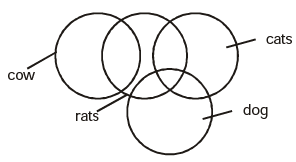Hence, some cats are rats is only conclusion that follows.

Question 19: Mr. ‘X’ has three children. The birthday of the first child falls on the 5th Monday of April, that of the second one falls on the 5th Thursday of November. On which day is the birthday of his third child, which falls on 20th December?    [2019-II]
(a) Monday
(b) Thursday
(c) Saturday
(d) Sunday

5th monday of April will fall on 29th or 30th April. again 5th thursday of November will fall on 29th or 30th November.
When 29th April is Monday.
then, Number of days between 29th April and 29th November = 214 days
= 30 weeks + 4 days.
thus 29th of November would be Friday.
But 29th November is thursday, hence, 30th April is Monday.
Now, Number of days between 29th November and 20th december = 21 days. = 3 weeks
Hence, 20th december is Thursday.

Question 20: Which year has the same calendar as that of 2009?    [2019-II]
(a) 2018
(b) 2017
(c) 2016
(d) 2015

Number of odd days in a non-Leap year = 1.

Number of odd days in a Leap year = 2.

Two year have same calender when number of odd days between two years is 7.

Between 2009 and 2015: Number of non-leap year = 5 = 5 odd days

Number of Leap year = 1 = 2 odd days Hence, 2015 have same calendar as 2009.

Question 21: Consider two statements S1 and S2 followed by a question:
S1 : p and q both are prime numbers.
S2 : p + q is an odd integer.
Question : Is pq an odd integer?
Which one of the following is correct?    [2019-II]

(a) S1 alone is sufficient to answer the question
(b) S2 alone is sufficient to answer the question
(c) Both S1 and S2 taken together are not sufficient to answer the question
(d) Both S1 and S2 are necessary to answer the question

From statement s2, (p + q) is an odd integers. i.e., p and q are not either even or odd simultaneously. so, p and q are even and odd respectively and vice-versa.
Hence, p.q is always odd is incorrect. p.q is always even.

Question 22: ‘A’ started from his house and walked 20 m towards East, where his friend ‘B’ joined him. They together walked 10 m in the same direction. Then ‘A’ turned left while ‘B’ turned right and travelled 2 m  and 8 m respectively. Again ‘B’ turned left to travel 4 m followed by 5 m to his right to reach his office. ‘A’ turned right and travelled 12 m to reach his office. What is the shortest distance between the two offices?    [2019-II]
(a) 15 m
(b) 17 m
(c) 19 m
(d) 20 m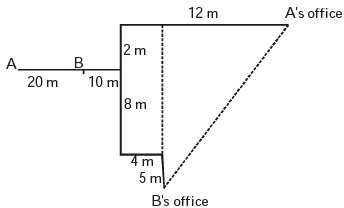Shortest distance between A and B.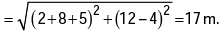Question 23: Consider the following sequence that follow some arrangement:
c_accaa_aa_bc_b
The letters that appear in the gaps are    [2019-II]
(a) abba
(b) cbbb
(c) bbbb
(d) cccc

The series is ccacc, aabaa, bbcbb

Question 24: What is X in the sequence
4, 196, 16, 144, 36, 100, 64, X?
(a) 48
(b) 64
(c) 125
(d) 256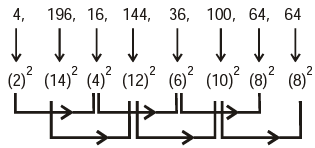This is double series sequence, here one series is increasing and other series is decreasing.

Question 25: If the numerator and denominator of a proper fraction are increased by the same positive quantity which is greater than zero, the resulting fraction is    [2019-II]
(a) always less than the original fraction
(b) always greater than the original fraction
(c) always equal to the original fraction
(d) such that nothing can be claimed definitely

A proper fraction is one which is less than 1. when same positive number is added in numerator and de-nominator then, fraction value increases from original value.
For example, when 1 is added to both numerator and denominator of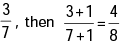Now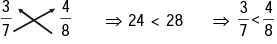Question 26: What is X in the sequence
132, 129, 124, 117, 106, 93, X?    [2019-II]
(a) 74
(b) 75
(c) 76
(d) 77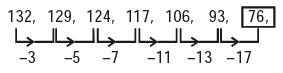Question 27: DIRECTIONS: Read the following statements S1 and S2 and answer the two items that follow:
S1 : Twice the weight of Sohan is less than the weight of Mohan or that of Rohan.
S2 : Twice the weight of Rohan is greater than the weight of Mohan or that of Sohan.

Which one of the following statements is correct?
(a) Weight of Mohan is greatest
(b) Weight of Sohan is greatest
(c) Weight of Rohan is greatest
(d) ‘Whose weight is greatest’ cannot be determined

S1 → 2 × (Sohan's weight) < {(Mohan's weight) or (Rohan's weight)}

S2 → 2 × (Rohan's weight) > {(Mohan's weight) or (Sohan's weight)}

From S1 and S2 , either Rohan or Mohan's weight is greatest but we can't determined whose weight is greatest.

Question 28: DIRECTIONS: Read the following statements S1 and S2 and answer the two items that follow:
S1 : Twice the weight of Sohan is less than the weight of Mohan or that of Rohan.
S2 : Twice the weight of Rohan is greater than the weight of Mohan or that of Sohan.

Which one of the following statements is correct?     [2019-II]
(a) Weight of Mohan is least
(b) Weight of Sohan is least
(c) Weight of Rohan is least
(d) ‘Whose weight is least’ cannot be determined

S1 → 2 × (Sohan's weight) < {(Mohan's weight) or (Rohan's weight)}

S2 → 2 × (Rohan's weight) > {(Mohan's weight) or (Sohan's weight)}

From S1, weight of Sohan is least.

Question 29: DIRECTIONS: Read the following information and answer the three items that follow:
Six students A, B, C, D, E and F appeared in several tests. Either C or F scores the highest. Whenever C scores the highest, then E scores the least, Whenever F scores the highest, B scores the least.
In all the tests they got different marks; D scores higher than A, but they are close competitors; A scores higher than B; C scores higher than A.

If F stands second in the ranking, then the position of B is    [2019-II]
(a) Third
(b) Fourth
(c) Fifth
(d) Sixth

Case - I: When C gets highest marks,

(i) E < F < B < A < D < C or,

(ii) E < B < F < A < D < C or,

(iii) E < B < A < D < F < C.

Case - II: When F Scores highest,

(i) B < A < D < C < E < F. or,

(ii) B < A < D < E < C < F.

From case I (iii), When F stand second B is fifth.

Question 30: DIRECTIONS: Read the following information and answer the three items that follow:
Six students A, B, C, D, E and F appeared in several tests. Either C or F scores the highest. Whenever C scores the highest, then E scores the least, Whenever F scores the highest, B scores the least.
In all the tests they got different marks; D scores higher than A, but they are close competitors; A scores higher than B; C scores higher than A.

If B scores the least, the rank of C will be    [2019-II]
(a) Second
(b) Third
(c) Fourth
(d) Second or third

Case - I: When C gets highest marks,

(i) E < F < B < A < D < C or,

(ii) E < B < F < A < D < C or,

(iii) E < B < A < D < F < C.

Case - II: When F Scores highest,

(i) B < A < D < C < E < F. or,

(ii) B < A < D < E < C < F.

From Case II, When B score the least then C stand either second or third.

Question 31: DIRECTIONS: Read the following information and answer the three items that follow:
Six students A, B, C, D, E and F appeared in several tests. Either C or F scores the highest. Whenever C scores the highest, then E scores the least, Whenever F scores the highest, B scores the least.
In all the tests they got different marks; D scores higher than A, but they are close competitors; A scores higher than B; C scores higher than A.

If E is ranked third, then which one of the following is correct?    [2019-II]
(a) E gets more marks than C
(b) C gets more marks than E
(c) A is ranked fourth
(d) D is ranked fifth

Case - I: When C gets highest marks,

(i) E < F < B < A < D < C or,

(ii) E < B < F < A < D < C or,

(iii) E < B < A < D < F < C.

Case - II: When F Scores highest,

(i) B < A < D < C < E < F. or,

(ii) B < A < D < E < C < F.

From case - II (ii), When E ranked third, then C score more then E.

Question 32: If every alternative letter of the English alphabet from B onwards (including B) is written in lower case (small letters) and the remaining letters are capitalized, then how is the first month of the second half of the year written?    [2019-II]
(a) JuLY
(b) jULy
(c) jUly
(d) jUlY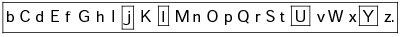Required answer is jUlY. Hence option (d) is correct.

Question 33: In the sequence 1, 5, 7, 3, 5, 7, 4, 3, 5, 7, how many such 5s are there which are not immediately preceded by 3 but are immediately followed by 7?    [2019-II]
(a)1
(b) 2
(c)3
(d) None

According to the question,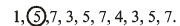Option (a) is correct.

Question 34: A joint family consists of seven members A, B, C, D, E, F and G with three females. G is a widow and sister-in-law of D’s father F, B and D are siblings and A is daughter of B. C is cousin of B. Who is E?    [2019-II]
(a) Wife of F
(b) Grandmother of A
(c) Aunt of C
Select the correct answer using the code given below:

(a) 1 and 2 only
(b) 2 and 3 only
(c) 1 and 3 only
(d) 1, 2 and 3

According to the question,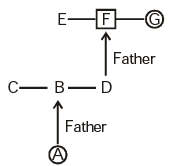So, E may be wife of F; Grandmother of A and Aunt of C
∴ Option (d) is correct.

Question 35: If \$ means ‘divided by’; @ means ‘multiplied by’; # means ‘minus’; then the value of 10#5@1\$5 is    [2019-II]
(a) 0
(b) 1
(c) 2
(d) 9

10 # 5 @ 1 \$ 5
= 10 – 5 × 1 ÷ 5 = 10 – 5 × (1/5) = 10 – 1 = 9.

Question 36: DIRECTIONS: Read the following information and answer the two items that follow.
The plan of an office block for six officers A, B,C, D, E and F is as follows : Both B and C occupy offices to the right of the corridor (as one enters the office block) and A occupies on the left of the corridor. E and F occupy offices on opposite sides of the Corridor but their offices do not face each other. The offices of  C and D face each other. E does not have a corner office. Fs office is further down the corridor than A’s, but on the same side.

If E sits in his office and faces the corridor, whose office is to his left?    [2018-II]
(a) A
(b) B
(c) C
(d) D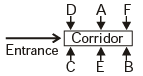Question 37: DIRECTIONS: Read the following information and answer the two items that follow.
The plan of an office block for six officers A, B,C, D, E and F is as follows : Both B and C occupy offices to the right of the corridor (as one enters the office block) and A occupies on the left of the corridor. E and F occupy offices on opposite sides of the Corridor but their offices do not face each other. The offices of  C and D face each other. E does not have a corner office. Fs office is further down the corridor than A’s, but on the same side.

Who is/are Fs immediate neighbour/neighbours?    [2018-II]
(a) A only
(b) A and D
(c) C only
(d) B and CQuestion 38: If LSJXVC is the code for MUMBAI, the code for DELHI is    [2018-II]
(a) CCIDD
(b) CDKGH
(c) CCJFG
(d) CCIFE

As,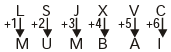Similarly,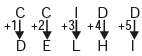Question 39: If RAMON is written as 12345 and DINESH as 675849, then HAMAM will be written as    [2018-II]
(a) 92233
(b) 92323
(c) 93322
(d) 93232

As,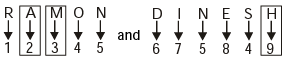Similarly,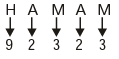Question 40: DIRECTIONS: Read the information given below and answer the six items that follow.
A, B, C and D are students. They are studying in four different cities, viz., P, Q, R and S (not necessarily in that order). They are studying in Science college, Arts college, Commerce college and Engineering college (not necessarily in that order), which are situated in four different States, viz., Gujarat, Rajasthan, Assam and Kerala (not necessarily in that order). Further, it is given that
(i) D is studying in Assam
(ii) Arts college is located in city S which is in Rajasthan
(iii) A is studying in Commerce college
(iv) B is studying in city Q
(v) Science college is located in Kerala

A is studying in    [2018-II]
(a) Rajasthan
(b) Gujarat
(c) city Q
(d) Kerala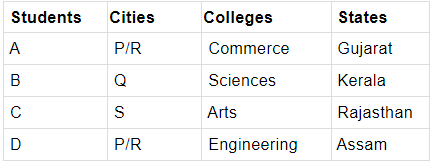The document Mental Ability, Analytical & Logical Reasoning- Solved Questions(1995-2020)- 1 Notes | Study UPSC Topic Wise Previous Year Questions - UPSC is a part of the UPSC Course UPSC Topic Wise Previous Year Questions.
All you need of UPSC at this link: UPSCUse Code STAYHOME200 and get INR 200 additional OFF

## UPSC Topic Wise Previous Year Questions

98 docs|91 tests

### How to Prepare for UPSC

Read our guide to prepare for UPSC which is created by Toppers & the best Teachers

Track your progress, build streaks, highlight & save important lessons and more!

,

,

,

,

,

,

,

,

,

,

,

,

,

,

,

,

,

,

,

,

,

,

,

,

;